• 編輯推薦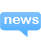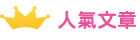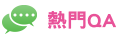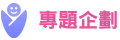{{LeftAD.Introduction}} more

• {{RigthAD1.Introduction}}

• {{RigthAD2.Introduction}}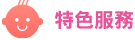{{adlist[currentindex].SubTitle}}
{{adlist[currentindex].Introduction}} more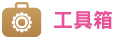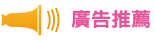{{adlist[currentindex].Introduction}} more{{adlist[currentindex].SubTitle}}
{{adlist[currentindex].Introduction}} more{{adlist[currentindex].Introduction}} more